# MATHEMATICS —2004 (Set II— Outside Delhi)

Except for the following questions, ali the remaining questions have been asked in Set I.

Q. 1. Using quadratic formula, solve the following quadratic equation for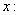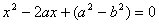Ans.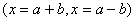Q. 2. If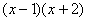is the GCD of the Polynomials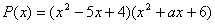, and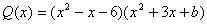find the values of a and b.

Ans.(a=5,b= -4)

Q. 6. The 8 th term of an Arithmetic Progression (A.P.) is -23 and its 12 th term is -39, Find the A.P.

Ans.(5,1,-3,-7,..)

Q. 7. A loan of Rs. 5,300 is to be returned in two equal annual instalments. If the rate of interest is 12% per annum, compounded annually, calculate the amount of each instalment.

Ans.(Rs. 3,136)

Q. 13. Determine the ratio in which the point (-6, a) divides the join of A(-3, -1) and B(-8,9). Also find the value of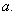Ans.(a=5)

Q. 20. A bag contains 3 red, 5 black and 7 white balls. A ball is drawn from the bag at random. Find the probability that the ball drawn is (i) white (ii) red (iii) not black (iv) red or white

Ans.(7/15,1/5,2/3,2/3)

Q. 23. The angles of elevation of the top of a tower from two points P and Q at distances of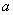and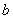respectively from the base and in the same straight line with it, are complementary. Prove that the height of the tower is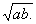Maths 2004 Question Papers Class X
CBSE 2004 Question Papers Class X